Next: Native Star Files Up: GCX User's Manual Previous: Noise Modelling   Contents

# Robust Averaging

A robust averaging algorithm is implemented by GCX and used in several places, most notably for zeropoint fitting by the aperture photometry and multiframe reduction routines. The algorithm calculates the robust average of a number of values (for the zeropoint routines, these are the differences between the standard and instrumental magnitudes of standard stars).

The data used consists of the values we want to calculate, and the estimated error of each value. For fitting frame zeropoints they are: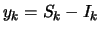(B.1)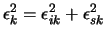(B.2)

where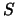is the standard magnitude,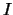is the instrumental magnitude,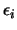is the estimated error of the instrumental magnitude,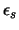is the error of the standard magnitude of each star. Each star is assigned a natural weight, calculated as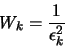(B.3)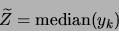(B.4)

and calculate the residuals of each value: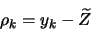(B.5)

and the standard errors: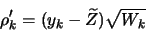(B.6)

The expected value of each standard error is 1. We can identify possible outliers by their large standard errors. A simple way to treat outliers is to just exclude from the fit any value that has a standard error larger than a certain threshold. This has the disadvantage that small changes in the values can cause large jumps in the solution if an outlier just crosses the threshold. Instead, we adjust the weights of the data points to reduce the outliers' contribution to the solution: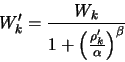(B.7)

The weighting function reduces the weight of values that have residuals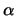times larger than expected to one half. Of course values with even larger residuals are downweighted even more. The parameter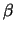tunes the sharpness'' of the weighting function.B.1A new estimate of the average is produced by: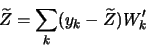(B.8)

The residual calculation, weighting and average estimating are iterated until the estimate doesn't change.

Finally, the error for the estimated parameters is calculated. the error of the zero point is:(B.9)

and the mean error of unit weight is: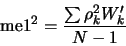(B.10)

where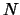is the number of standard stars. The mean error of unit weight is 1 in the ideal case (when all the errors are estimated correctly). A significantly larger value should raise doubts about the error estimates.Next: Native Star Files Up: GCX User's Manual Previous: Noise Modelling   Contents